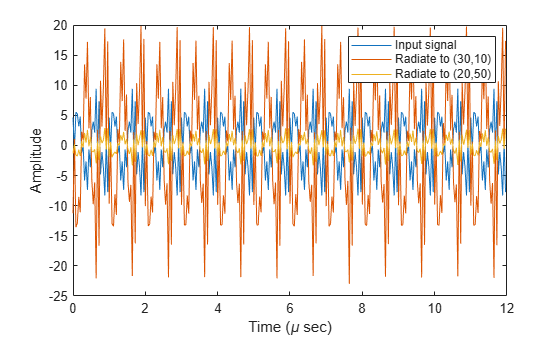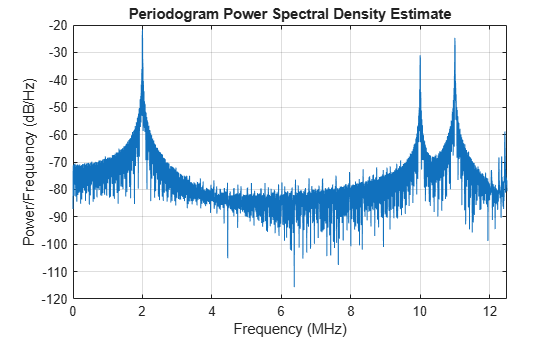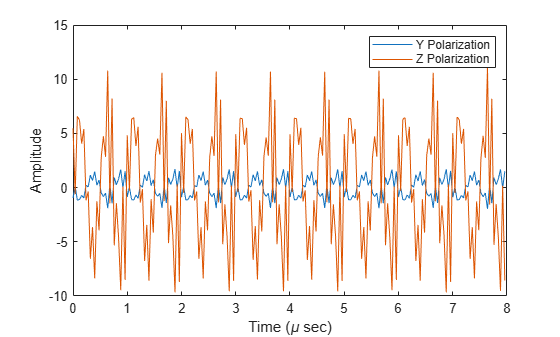## Description

The phased.WidebandRadiator System object™ implements a wideband signal radiator. A radiator converts signals into radiated wavefields transmitted from arrays and individual sensor elements such as antennas, microphone elements, and sonar transducers. The radiator output represents the fields at a reference distance of one meter from the phase center of the element or array. The algorithm divides the signal at each element into frequency subbands and applies a narrowband time-delay to each signal using the phase-shift approximation. Then, the delayed subbands are coherently added to create the output signal. You can then propagate the signals to the far field using, for example, the phased.WidebandFreeSpace, phased.WidebandLOSChannel, or phased.WidebandTwoRayChannel System objects. You can use this object to

• model radiated signals as polarized or non-polarized fields depending upon whether the element or array supports polarization and the value of the Polarization property. Using polarization, you can transmit a signal as a polarized electromagnetic field, or transmit two independent signals using dual polarizations.

• model acoustic radiated fields by using nonpolarized microphone and sonar transducer array elements and by setting the Polarization to 'None'. You must also set the PropagationSpeed to a value appropriate for the medium.

• radiate fields from subarrays created by the phased.ReplicatedSubarray and phased.PartitionedArray objects. You can steer all subarrays in the same direction using the Steering angle argument, STEERANG, or steer each subarray in a different direction using the Subarray element weights argument, WS. The radiator distributes the signal powers equally among the elements of each subarray.

1. Create the phased.WidebandRadiator object and set its properties.

2. Call the object with arguments, as if it were a function.

## Creation

### Syntax

radiator = phased.WidebandRadiator
radiator = phased.WidebandRadiator(Name,Value)

### Description

radiator = phased.WidebandRadiator creates a wideband signal radiator object, radiator, with default property values.
radiator = phased.WidebandRadiator(Name,Value) creates a wideband signal radiator with each property Name set to a specified Value. You can specify additional name-value pair arguments in any order as (Name1,Value1,...,NameN,ValueN). Enclose each property name in single quotes.Example: radiator = phased.WidebandRadiator('Sensor',phased.URA,'CarrierFrequency',300e6) sets the sensor array to a uniform rectangular array (URA) with default URA property values. The beamformer has a carrier frequency of 300 MHz.

## Properties

expand all

Unless otherwise indicated, properties are nontunable, which means you cannot change their values after calling the object. Objects lock when you call them, and the release function unlocks them.

If a property is tunable, you can change its value at any time.

Sensor element or sensor array, specified as a System object belonging to Phased Array System Toolbox. A sensor array can contain subarrays.

Example: phased.URA

Signal propagation speed, specified as a positive scalar. Units are in meters per second. The default propagation speed is the value returned by physconst('LightSpeed'). See physconst for more information.

Example: 3e8

Data Types: double

Signal sample rate, specified as a positive real-valued scalar. Units are in hertz.

Example: 1e6

Data Types: double

Carrier frequency, specified as a positive real-valued scalar. Units are in hertz.

Example: 1e6

Data Types: double

Number of processing subbands, specified as a positive integer.

Example: 128

Data Types: double

Sensor gain measure, specified as 'dB' or 'dBi'.

• When you set this property to 'dB', the input signal power is scaled by the sensor power pattern (in dB) at the corresponding direction and then combined.

• When you set this property to 'dBi', the input signal power is scaled by the directivity pattern (in dBi) at the corresponding direction and then combined. This option is useful when you want to compare results with the values computed by the radar equation that uses dBi to specify the antenna gain. The computation using the 'dBi' option is expensive as it requires an integration over all directions to compute the total radiated power of the sensor.

Data Types: char

Polarization configuration, specified as 'None', 'Combined', or 'Dual'. When you set this property to 'None', the output field is considered a scalar field. When you set this property to 'Combined', the radiated fields are polarized and are interpreted as a single signal in the sensor's inherent polarization. When you set this property to 'Dual', the H and V polarization components of the radiated field are independent signals.

Example: 'Dual'

Data Types: char

Enable weights input, specified as false or true. When true, use the object input argument W to specify weights. Weights are applied to individual array elements (or at the subarray level when subarrays are supported).

Data Types: logical

## Usage

### Syntax

Y = radiator(X,ANG)
Y = radiator(X,ANG,LAXES)
Y = radiator(XH,XV,ANG,LAXES)
Y = radiator(___,W)
Y = radiator(___,STEERANG)
Y = radiator(___,WS)
Y = radiator(X,ANG,LAXES,W,STEERANG)

### Description

example

Y = radiator(X,ANG) radiates the signal X in the directions specified by ANG. For each direction, the method computes the radiated signal, Y, by summing the contributions of each element or subarray.

example

Y = radiator(X,ANG,LAXES) also specifies the local coordinate system of the radiator, LAXES. This syntax applies when you set the Polarization property to 'Combined'.
Y = radiator(XH,XV,ANG,LAXES) specifies a horizontal-polarization port signal, XH, and a vertical-polarization port signal, XV. To use this syntax, set the Polarization property to 'Dual'.
Y = radiator(___,W) also specifies W as array element or subarray weights. To use this syntax, set the WeightsInputPort property to true.
Y = radiator(___,STEERANG) also specifies STEERANG as the subarray steering angle. To use this syntax, set the Sensor property to an array that supports subarrays and set the SubarraySteering property of that array to either 'Phase' or 'Time'.
Y = radiator(___,WS) also specifies WS as the weights applied to each element within each subarray. To use this syntax, set the Sensor property to an array that supports subarrays and set the SubarraySteering of that array to 'Custom'.
You can combine optional input arguments when their enabling properties are set, for example, Y = radiator(X,ANG,LAXES,W,STEERANG) combines several input arguments. Optional inputs must be listed in the same order as the order of the enabling properties.

### Input Arguments

expand all

Signal to radiate, specified as a complex-valued M-by-1 vector or complex-valued M-by-N matrix. M is the length of the signal, and N is the number of array elements (or subarrays when subarrays are supported).

Dimensions of X

DimensionSignal
M-by-1 vector

The same signal is radiated from all array elements (or all subarrays when subarrays are supported).

M-by-N matrixEach column corresponds to the signal radiated by the corresponding array element (or corresponding subarrays when subarrays are supported).

The size of the first dimension of the input matrix can vary to simulate a changing signal length. A size change can occur, for example, in the case of a pulse waveform with variable pulse repetition frequency.

#### Dependencies

To enable this argument, set the Polarization property to 'None' or 'Combined'.

Data Types: double
Complex Number Support: Yes

Radiating directions of signals, specified as a real-valued 2-by-L matrix. Each column specifies a radiating direction in the form [AzimuthAngle;ElevationAngle]. The azimuth angle must lie between –180° and 180°, inclusive. The elevation angle must lie between –90° and 90°, inclusive. Units are in degrees.

Example: [30,20;45,0]

Data Types: double

Local coordinate system, specified as a real-valued 3-by-3 orthogonal matrix. The matrix columns specify the local coordinate system's orthonormal x, y, and z axes with respect to the global coordinate system.

Example: rotx(30)

#### Dependencies

To enable this argument, set the Polarization property to 'Combined' or 'Dual'.

Data Types: double

H-polarization port signal to radiate, specified as a complex-valued M-by-1 vector or complex-valued M-by-N matrix. M is the length of the signal, and N is the number of array elements (or subarrays when subarrays are supported).

Dimensions of XH

DimensionSignal
M-by-1 vector

The same signal is radiated from all array elements (or all subarrays when subarrays are supported).

M-by-N matrixEach column corresponds to the signal radiated by the corresponding array element (or corresponding subarrays when subarrays are supported).

The dimensions and sizes of XH and XV must be the same.

The size of the first dimension of the input matrix can vary to simulate a changing signal length. A size change can occur, for example, in the case of a pulse waveform with variable pulse repetition frequency.

#### Dependencies

To enable this argument, set the Polarization property to 'Dual'.

Data Types: double
Complex Number Support: Yes

V-polarization port signal to radiate, specified as a complex-valued M-by-1 vector or complex-valued M-by-N matrix. M is the length of the signal, and N is the number of array elements (or subarrays when subarrays are supported).

Dimensions of XV

DimensionSignal
M-by-1 vector

The same signal is radiated from all array elements (or all subarrays when subarrays are supported).

M-by-N matrixEach column corresponds to the signal radiated by the corresponding array element (or corresponding subarrays when subarrays are supported).

The dimensions and sizes of XH and XV must be the same.

The size of the first dimension of the input matrix can vary to simulate a changing signal length. A size change can occur, for example, in the case of a pulse waveform with variable pulse repetition frequency.

#### Dependencies

To enable this argument, set the Polarization property to 'Dual'.

Data Types: double
Complex Number Support: Yes

Element or subarray weights, specified as a complex-valued N-by-1 column vector where N is the number of array elements (or subarrays when the array supports subarrays).

#### Dependencies

To enable this argument, set the WeightsInputPort property to true.

Data Types: double
Complex Number Support: Yes

Subarray element weights, specified as complex-valued NSE-by-N matrix or 1-by-N cell array where N is the number of subarrays. These weights are applied to the individual elements within a subarray.

Subarray element weights

Sensor ArraySubarray weights
phased.ReplicatedSubarray

All subarrays have the same dimensions and sizes. Then, the subarray weights form an NSE-by-N matrix. NSE is the number of elements in each subarray and N is the number of subarrays. Each column of WS specifies the weights for the corresponding subarray.

phased.PartitionedArray

Subarrays may not have the same dimensions and sizes. In this case, you can specify subarray weights as

• an NSE-by-N matrix, where NSE is now the number of elements in the largest subarray. The first Q entries in each column are the element weights for the subarray where Q is the number of elements in the subarray.

• a 1-by-N cell array. Each cell contains a column vector of weights for the corresponding subarray. The column vectors have lengths equal to the number of elements in the corresponding subarray.

#### Dependencies

To enable this argument, set the Sensor property to an array that contains subarrays and set the SubarraySteering property of the array to 'Custom'.

Data Types: double
Complex Number Support: Yes

Subarray steering angle, specified as a length-2 column vector. The vector has the form [azimuthAngle;elevationAngle]. The azimuth angle must be between –180° and 180°, inclusive. The elevation angle must be between –90° and 90°, inclusive. Units are in degrees.

Example: [20;15]

#### Dependencies

To enable this argument, set the Sensor property to an array that supports subarrays and set the SubarraySteering property of that array to either 'Phase' or 'Time'

Data Types: double

### Output Arguments

expand all

Radiated signals, specified as a complex-valued M-by-L matrix or a 1-by-L cell array, where L is the number of radiating angles, ANG. M is the length of the input signal, X.

• If the Polarization property value is set to 'None', the output argument Y is an M-by-L matrix.

• If the Polarization property value is set to 'Combined' or 'Dual', Y is a 1-by-L cell array of structures. Each cell corresponds to a separate radiating signal. Each struct contains three column vectors containing the X, Y, and Z components of the polarized fields defined with respect to the global coordinate system.

Data Types: double
Complex Number Support: Yes

## Object Functions

To use an object function, specify the System object as the first input argument. For example, to release system resources of a System object named obj, use this syntax:

release(obj)

expand all

 step Run System object algorithm release Release resources and allow changes to System object property values and input characteristics reset Reset internal states of System object

## Examples

collapse all

Create a 5-by-5 URA and space the elements one-half wavelength apart. The wavelength corresponds to a design frequency of 300 MHz.

Note: This example runs only in R2016b or later. If you are using an earlier release, replace each call to the function with the equivalent step syntax. For example, replace myObject(x) with step(myObject,x).

Create 5-by-5 URA Array of Cosine Elements

c = physconst('LightSpeed'); fc = 100e6; lam = c/fc; antenna = phased.CosineAntennaElement('CosinePower',[2,2]); array = phased.URA('Element',antenna,'Size',[5,5],'ElementSpacing',[0.5,0.5]*lam);

Radiate a wideband signal consisting of three sinusoids at 2, 10 and 11 MHz. Set the sampling rate to 25 MHz. Radiate the fields into two directions: (30,10) degrees azimuth and elevation and (20,50) degrees azimuth and elevation.

fs = 25e6; f1 = 2e6; f2 = 10e6; f3 = 11e6; dt = 1/fs; Tsig = 100e-6; t = [0:dt:Tsig]; sig = 5.0*sin(2*pi*f1*t) + 2.0*sin(2*pi*f2*t + pi/10) + 4*sin(2*pi*f3*t + pi/2); radiatingangles = [30 10; 20 50]'; radiator = phased.WidebandRadiator('Sensor',array,'CarrierFrequency',fc,'SampleRate',fs); radsig = radiator(sig.',radiatingangles);

plot(t(1:300)*1e6,real(sig(1:300))) hold on plot(t(1:300)*1e6,real(radsig(1:300,1))) plot(t(1:300)*1e6,real(radsig(1:300,2))) hold off xlabel('Time (\mu sec)') ylabel('Amplitude') legend('Input signal','Radiate to (30,10)','Radiate to (20,50)')Plot the spectra of the signal that is radiated to (30,10) degrees.

periodogram(real(radsig(:,1)),rectwin(size(radsig,1)),4096,fs);Examine the polarized field produced by the wideband radiator from a five-element uniform line array (ULA) composed of short-dipole antenna elements.

Note: This example runs only in R2016b or later. If you are using an earlier release, replace each call to the function with the equivalent step syntax. For example, replace myObject(x) with step(myObject,x).

Set up the ULA of five short-dipole antennas with polarization enabled. The element spacing is set to 1/2 wavelength of the carrier frequency. Construct the wideband radiator System object(TM).

fc = 100e6; c = physconst('LightSpeed'); lam = c/fc; antenna = phased.ShortDipoleAntennaElement; array = phased.ULA('Element',antenna,'NumElements',5,'ElementSpacing',lam/2);

Radiate a signal consisting of the sum of three sine waves. Radiate the signal into two different directions. Radiated angles are azimuth and elevation angles defined with respect to a local coordinate system. The local coordinate system is defined by 10 degree rotation around the x-axis from the global coordinates.

fs = 25e6; f1 = 2e6; f2 = 10e6; f3 = 11e6; dt = 1/fs; fc = 100e6; t = [0:dt:100e-6]; sig = 5.0*sin(2*pi*f1*t) + 2.0*sin(2*pi*f2*t + pi/10) + 4*sin(2*pi*f3*t + pi/2); radiatingAngle = [30 30; 0 20]; laxes = rotx(10); radiator = phased.WidebandRadiator('Sensor',array,'SampleRate',fs,... 'CarrierFrequency',fc,'Polarization','Combined'); y = radiator(sig.',radiatingAngle,laxes);

Plot the first 200 samples of the y and z components of the polarized field propagating in the [30,0] direction.

plot(10^6*t(1:200),real(y(1).Y(1:200))) hold on plot(10^6*t(1:200),real(y(1).Z(1:200))) hold off xlabel('Time (\mu sec)') ylabel('Amplitude') legend('Y Polarization','Z Polarization')expand all